Part 3: How to use Macro to Trigger Excel Solver to Calculate Wet-Bulb Temperature from Tdb and RH

This page is a continuation for Advanced Psychrometric Calculator, which aims to provide explanation in regard to setting up excel solver to compute air properties, and linking some of the functions to more user-friendly control buttons.

The following computation method for Twb and RH is developed by Al-Ismaili and Al-Azri (2016) and further elaborated by Dr. John A. Knox. The computational model can be performed using Excel with its Solver function.

The Excel file, together with other computational methods for all Air Properties, can be downloaded from below. Take note that this has to be a Macro-Enabled Excel, and iteration shall be enabled for certain computation to take place.

Additionally, the following blog explains generally how to link a Solver to be triggered by a Macro-linked Button, also explained by Jacob Fairclough.

Firstly, Go to File – Options – Add-ins – Excel Add-Ins – Go – Select all the available Add-ins – and then click “OK”.

Form Controls

Check all the available add-ins, and then click “OK”. We want to make sure all 4 options are selected.Then, also in Options, go to Customise Ribbon and then check “Developer”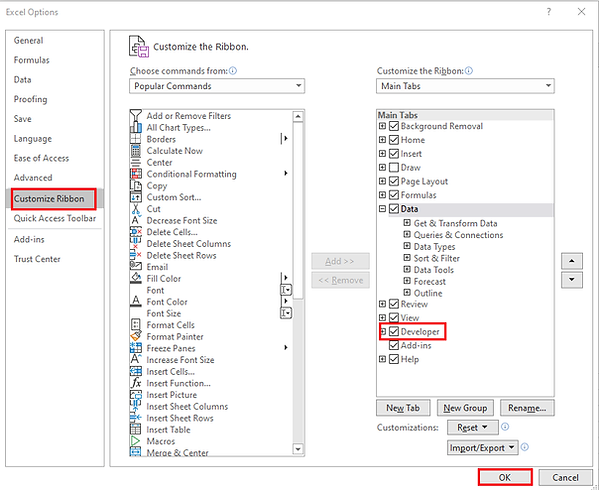We will see a Developer Ribbon coming up as soon as we click “OK”. Click on the “Developer” tab, and then click “Record Macro”.Give the Macro a name and/or a shortcut key to trigger the Macro to run

Once the recording is started, perform the steps to solve our parameter (Twb) using the Solver function. To simplify, we want to use the solver to solve for Twb, by changing the value of Twb by iteration, until the 2 values of Humidity Ratio (Cell C23 and C24) equal to one another. This is signified by a target cell set to be the difference between the 2 Values, where the Excel recognises it as “zero” when the target is met (C23 = C24; or C23-C24 = 0)

Click Solve, and then OK to accept the solver result. Then, we go to the Developer Tab again and “Stop Recording”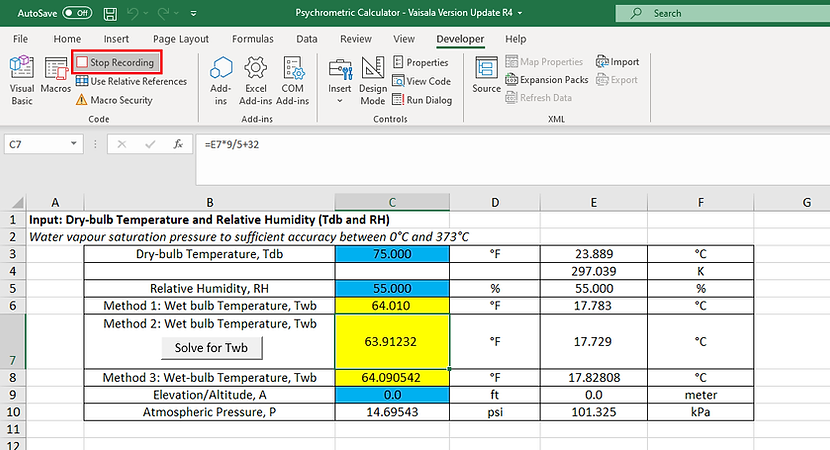The recorded Macro can be traced back from “Macros”. Click “Run” or use the assigned shortcut key to run the code; or “Edit” to edit the code.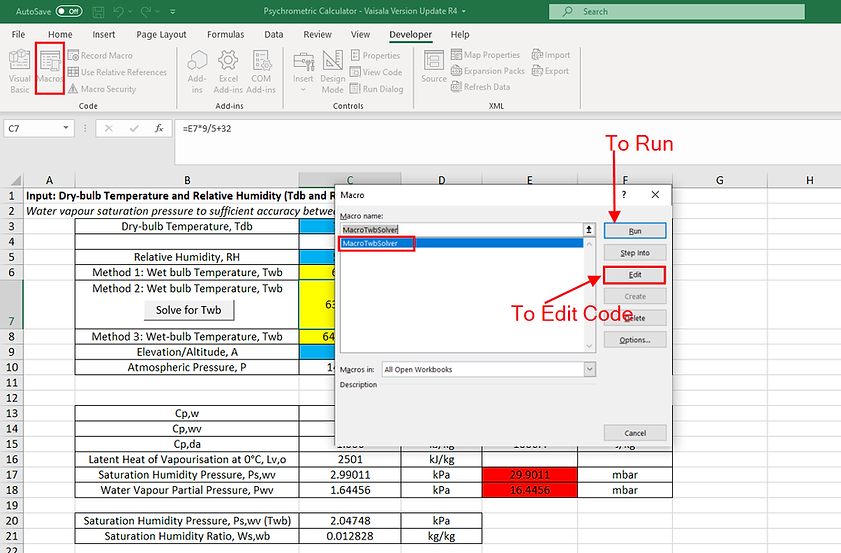Before we can run the code, there is one more step to do. Upon clicking “Edit”, you will be redirected to the VBA interface, with your recorded Macro translated into editable lines of codes.

Look for the available References and check “Solver”. If there is no such option, we need to make sure the 4 add-ins as mentioned just now were checked. Go to Options – Add-in, and then check all 4 solver related Add-ins from the list.

Last but not least, add in the word “(True)” at the end of SolverSolve, as shown below. This is to prevent the windows keep popping up, asking whether you would like to accept the solution provided by the Solver.Then, click “Run” from the VBA, or go back to Excel and use the assigned shortcut to run the Macro.We usually would like to make a button to be linked to the Macro. Go to Developer – Inset – Then select the Button IconSelect where you want the button to be, and then Assign a Macro to the buttonYou may edit the text appearance, move, resize, or assign another Macro to the button by right-clicking it. Left-clicking the button runs the assigned Macro. Alternatively, toggling the “Design Mode” Button enables and restrict selection of buttons for editing.The same steps is being repeated for the computation of Relative Humidity (RH) from Tdb and Twb

The only difference being, now, we iterate the RH (instead of Twb) repeatedly until we match the 2 values of Humidity Ratio, HR as depicted in the Target Cell (W1-W2). Our objective we set for the solver is still W1=W2, or as described by the target cell (W1-W2= 0).

2 things to take note:

1. For security purposes, Excel Macros may be disabled by default. As mentioned, we will need to enable Macros for the above functions to take place. If you ever doubt the trustability of a Macro, it is the safest to develop one on your own (with the guide above)

2. The Coding derived from the Recording of the Macro is specific for designated cells involved in the Solver. Any updates in the cell locations (such as adding or removing a row or column) would require the code to be updated (or re-recorded).

Part 4: Perform Unit Conversions for Excel

There are generally 2 methods to initiate the execution of codes in Excel, through a Form Control, or ActiveX Controls. While ActiveX Controls may be more efficient to perform complex operations, it has downsides in terms of stability, whose functionality may even be affected by outdated libraries in older versions of Excel. For enhanced stability, our Excel attached above (Revision R32) is utilising Form Controls’ Buttons. We also attach a version below (Revision R28) in which we use ActiveX Controls as a reference.

Method 1: Form Controls

We can insert a Form Controls’ option button anywhere in the workbook.

ActiveX Controls

Then, Right-Click the option button, and then click “Format Control”

In the “Control” Tab, select the reference cell where we want to link to the radio option button. In this configuration, when the “Imperial” radio button is checked, the reference cell “G2” will return “2”. Otherwise, it returns “1”.

These numbers can be very useful in further “manipulating” the result. For the example below, both imperial and metric units were displayed in different columns (to be hidden). The result-column relays the cells with the user’s selected unit based on the reference cell values (True/False), through a simple “IF-ELSE” function.Additionally, we can even add a code to toggle user-input values between “Imperial” and “Metric” units. A toggle button can be added and assigned to toggle input values between “Imperial” and “Metric" Units. For example, when the user enters 25 °C while “Metric” unit is selected, the value automatically converts to 77 °F as soon as the selection toggles to “Imperial” unit. (Code shown below):

Sub OptionButton22_Click() 'Metric Button

If Range("E2") < 0 Then

Range("G3").Value = Range("G3").Value

Range("G6").Value = Range("G6").Value

Range("G79").Value = Range("G79").Value

Range("G81").Value = Range("G81").Value

Range("G83").Value = Range("G83").Value

Else

Range("G3").Value = (Range("G3").Value - 32) * 5 / 9

Range("G6").Value = Range("G6").Value / 3.28084

Range("G79").Value = (Range("G79").Value - 32) * 5 / 9

Range("G81").Value = (Range("G81").Value - 32) * 5 / 9

Range("G83").Value = Range("G83").Value / 3.28084

Range("E2") = Range("E2") * -1

End If

End Sub

Sub OptionButton23_Click() 'Imperial Button

If Range("E2") > 0 Then

Range("G3").Value = Range("G3").Value

Range("G6").Value = Range("G6").Value

Range("G79").Value = Range("G79").Value

Range("G81").Value = Range("G81").Value

Range("G83").Value = Range("G83").Value

Else

Range("G3").Value = Range("G3").Value * 9 / 5 + 32

Range("G6").Value = Range("G6").Value * 3.28084

Range("G79").Value = Range("G79").Value * 9 / 5 + 32

Range("G81").Value = Range("G81").Value * 9 / 5 + 32

Range("G83").Value = Range("G83").Value * 3.28084

Range("E2") = Range("E2") * -1

End If

End Sub

The If-Else function and the other Reference Cell “E2” prevents Excel VBA to execute the conversion repeatedly if the button is selected again and again. (This reference cell toggles between 1 and -1 each time the button is clicked, and serves to rule of repeated button activation). In other words, we only want the function of conversion to execute once, not again and again if the same option is being registered for multiple times. The conversion function is to resume execution only for the first time the user toggles from another option. A tree-diagram best explains how the code is intended to work.An example of chain of actions (top right of photo above) shows that this tree-diagram gives us the result we want – to execute conversion only for the first time the user toggles from the other unit.

Method 2: ActiveX Controls

We can insert an ActiveX Controls’ Toggle Button anywhere in the workbook.As shown below, by holding down the “Design Mode” button, we can right click the Button to “edit property”. On the property panel, we can link the action of “toggling” of the Button as a “True” and “False” result in any desired linked reference cell. The reference cell will return “True” when the toggle button is pressed, and “False if Otherwise”.This is particularly simple and helpful for functions such as toggling between unit conversions (such as between Metric and Imperial Units). For the example below, both imperial and metric units were displayed in different columns (to be hidden). The result-column relays the cells with the user’s selected unit based on the reference cell values (True/False), through a simple “IF-ELSE” function.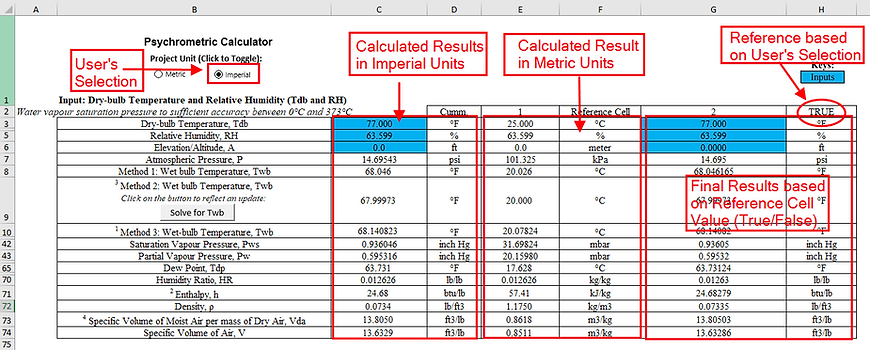Additionally, we can even add a code to toggle user-input values between “Imperial” and “Metric” units. A toggle button can be added and assigned to toggle input values between “Imperial” and “Metric Unit” (Code shown below):

Private Sub ToggleButton3_Click()

If ToggleButton3.Value = True Then

'This area contains the things you want to happen

'when the toggle button is not depressed

DoEvents

ToggleButton3.Caption = "Imperial"

DoEvents

Range("G3").Value = Range("G3").Value * 9 / 5 + 32

Range("G6").Value = Range("G6").Value * 3.28084

Range("G79").Value = Range("G79").Value * 9 / 5 + 32

Range("G81").Value = Range("G81").Value * 9 / 5 + 32

Range("G83").Value = Range("G83").Value * 3.28084

Else

'This area contains the things you want to happen

'when the toggle button is depressed

DoEvents

ToggleButton3.Caption = "Metric"

DoEvents

Range("G3").Value = (Range("G3").Value - 32) * 5 / 9

Range("G6").Value = Range("G6").Value / 3.28084

Range("G79").Value = (Range("G79").Value - 32) * 5 / 9

Range("G81").Value = (Range("G81").Value - 32) * 5 / 9

Range("G83").Value = Range("G83").Value / 3.28084

End If

End Sub

Part 5: Using Buttons to Hide/Unhide Rows and Columns

Steps for adding buttons are similar to those in Part 4. There are also 2 methods to initiate the execution of codes in Excel, through a Form Control, or ActiveX Controls.

Method 1: Form Controls

The use of Form Controls’ Toggle button to hide or unhide rows/columns is best described in the video shown by Guido’s How-to’s.

The 2 sub-codes below are 2 Macros linked to 2 separate Form Controls’ buttons to hide/unhide Method 2 & 3. These codes effectively toggle between hiding and unhiding desired rows defined in the Excel VBA.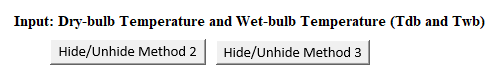Sub HideUnhideMethod2() 'Hide or Unhide Method 2

Rows("133:133").Hidden = Not Rows("133:133").Hidden

Rows("148:148").Hidden = Not Rows("148:148").Hidden

Rows("155:155").Hidden = Not Rows("155:155").Hidden

Rows("157:157").Hidden = Not Rows("157:157").Hidden

Rows("159:159").Hidden = Not Rows("159:159").Hidden

Rows("161:161").Hidden = Not Rows("161:161").Hidden

Rows("163:163").Hidden = Not Rows("163:163").Hidden

End Sub

Sub HideUnhideMethod3() 'Hide or Unhide Method 3

Rows("134:134").Hidden = Not Rows("134:134").Hidden

Rows("149:149").Hidden = Not Rows("149:149").Hidden

Rows("156:156").Hidden = Not Rows("156:156").Hidden

Rows("158:158").Hidden = Not Rows("158:158").Hidden

Rows("160:160").Hidden = Not Rows("160:160").Hidden

Rows("162:162").Hidden = Not Rows("162:162").Hidden

Rows("164:164").Hidden = Not Rows("164:164").Hidden

End Sub

Method 2: ActiveX Controls

The ActiveX Controls’ Toggle button is also helpful to perform hiding or unhiding of rows or columns.

https://best-excel-tutorial.com/57-vba-tutorial/243-toggle-button

The 2 sub-codes below linked to 2 separate ActiveX Controls’ buttons to hide/unhide Method 2 & 3 in our Excel File. These codes effectively toggle between hiding and unhiding desired rows defined in the Excel VBA.

Private Sub ToggleButton1_Click() 'Hide or Unhide Method 2

If ToggleButton1.Value = True Then

'This area contains the things you want to happen

'when the toggle button is not depressed

Rows(133).EntireRow.Hidden = True

Rows(148).EntireRow.Hidden = True

Rows(155).EntireRow.Hidden = True

Rows(157).EntireRow.Hidden = True

Rows(159).EntireRow.Hidden = True

Rows(161).EntireRow.Hidden = True

Rows(163).EntireRow.Hidden = True

ToggleButton1.Caption = "Show Method 2"

Else

'This area contains the things you want to happen

'when the toggle button is depressed

Rows(133).EntireRow.Hidden = False

Rows(148).EntireRow.Hidden = False

Rows(155).EntireRow.Hidden = False

Rows(157).EntireRow.Hidden = False

Rows(159).EntireRow.Hidden = False

Rows(161).EntireRow.Hidden = False

Rows(163).EntireRow.Hidden = False

ToggleButton1.Caption = "Hide Method 2"

End If

End Sub

Private Sub ToggleButton2_Click() 'Hide or Unhide Method 3

If ToggleButton2.Value = True Then

'This area contains the things you want to happen

'when the toggle button is not depressed

Rows(134).EntireRow.Hidden = True

Rows(149).EntireRow.Hidden = True

Rows(156).EntireRow.Hidden = True

Rows(158).EntireRow.Hidden = True

Rows(160).EntireRow.Hidden = True

Rows(162).EntireRow.Hidden = True

Rows(164).EntireRow.Hidden = True

ToggleButton2.Caption = "Show Method 3"

Else

'This area contains the things you want to happen

'when the toggle button is depressed

Rows(134).EntireRow.Hidden = False

Rows(149).EntireRow.Hidden = False

Rows(156).EntireRow.Hidden = False

Rows(158).EntireRow.Hidden = False

Rows(160).EntireRow.Hidden = False

Rows(162).EntireRow.Hidden = False

Rows(164).EntireRow.Hidden = False

ToggleButton2.Caption = "Hide Method 3"

End If

End Sub